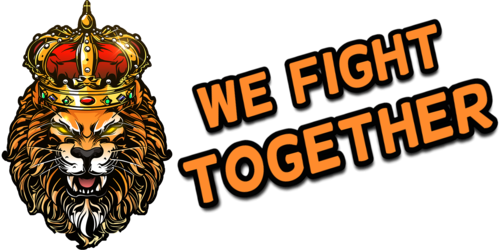search
0# test (from =WFT= Dutch Gaming Clan / Community Voor Squad, Post

januari 1, 1970Uncategorized

=09
=09
=09
=
=09
=09
=09test (from=
=3DWFT=3D Dutch Gaming Clan / Community Voor Squad, Post Scriptum & Ar=
ma 3.)

=

=09
=09=09
=09=09
=09=09=09
=09=09=09=09=3DWFT=3D Dutch G=
aming Clan / Community Voor Squad, Post Scriptum & Arma 3.
=09=09=
=09
=09=09
=09=09
=09=09=09

The following m=
essage has been sent from =3DWFT=3D RobinLollig <robin=
[email protected]> (IP: 185.200.132.211) via the contact form at =3DWFT=3D Dutch Gaming Clan / Community Voor Squad, Post Scriptu=
m & Arma 3..

test

test=

=09=09=09
=09=09
=09=09
=09=09=09
=09=09=09=09

Visit =3DWFT=3D Dutch Gaming Clan / Community Voor Squad, Post=
Scriptum & Arma 3.

=09=09=09=09
=09=09=09
=09=
=09
=09=09
=09